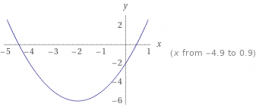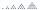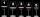# Three unknown numbers

The sum of of three numbers is 106. the first number is 10 more then the third. The second number is 2x the third. What are the numbers?

a =  34
b =  48
c =  24

### Step-by-step explanation:

a+b+c=106
a=10+c
b=2•c

a+b+c = 106
a-c = 10
b-2c = 0

a = 34
b = 48
c = 24

Our linear equations calculator calculates it.Did you find an error or inaccuracy? Feel free to write us. Thank you!Tips to related online calculators
Do you have a system of equations and looking for calculator system of linear equations?

## Related math problems and questions:

• Two numbersWe have two numbers. Their sum is 140. One-fifth of the first number is equal to half the second number. Determine those unknown numbers.
• The sumThe sum of the first 10 members of the arithmetic sequence is 120. What will be the sum if the difference is reduced by 2?
• Two unknown numbersOne number is 2 less than a second number. Twice the second number is 14 less than 5 times the first. Find the two numbers.
• Four numbersThe first number is 50% second, the second number is 40% third, the third number is 20% of the fourth. The sum is 396. What are the numbers?
• Unknown number 10Number first increased by 30%, then by 1/5. What percentage we've increased the original number?
• Find the sumFind the sum of all natural numbers from 1 and 100, which are divisible by 2 or 5
• Two numbersThe sum of two numbers is 1. Identify this two numbers if you know that the half of first is equal to the third of second number.Divide number 135 into two additions so that one adds 30 more than 2/5 of the other add. Write the bigger one.
• Three workshopsThere are 2743 people working in three workshops. In the second workshop works 140 people more than in the first and in third works 4.2 times more than the second one. How many people work in each workshop?
• Three numbersFind three numbers so that the second number is 4 times greater than the first and the third is lower by 5 than the second number. Their sum is 67.
• The HotelThe Holiday Hotel has the same number of rooms on each floor. Rooms are numbered with natural numerals sequentially from the first floor, no number is omitted, and each room has a different number. Three tourists arrived at the hotel. The first one was in
• Sum and ratioThe sum and ratio of two numbers is equal to 10. Which numbers are they?
• Boxes200 boxes have been straightened in three rows. The first was 13 more than in the second, and in the second was one fifth more than in the third one. How many boxes are in each row?
• Three numbersWhat are three numbers that have the property: the sum of the first and second numbers' reciprocals is 12/7, the first and third 11/24, and the second and the third 3/8.
• The sumThe sum of the squares of two immediately following natural numbers is 1201. Find these numbers.
• 925 USDFour classmates saved an annual total 925 USD. The second save twice as the first, third 35 USD more than the second and fourth 10 USD less than the first. How USD save each of them?
• CupsWe have three cups. In the cups we had fluid and boredom we started to shed. 1 We shed one-third of the fluid from the second glass into the first and third. 2 Then we shed one quarter cup of liquid from the first to the second and to the third. 3 Then we